# Visual Tracking

The ideas introduced in this tutorial have not been verified to be according to FIRST rules. Please verify with the rule manual on any modifications or unique usage of items provided in the FIRST kit of parts.

This tutorial assumes that you have acquired a visual target but need to remove your robot or turret to be inline with the target in order to shoot.

Let's assume the following two images are being viewed. They show the target at different locations in the image plane which would require different actions of the robot or turret in order to get then aligned.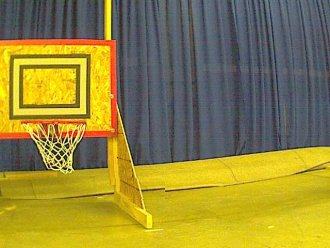Using the results from the Tracking Tutorial we have the following results.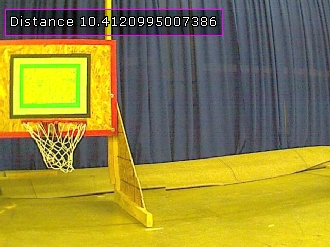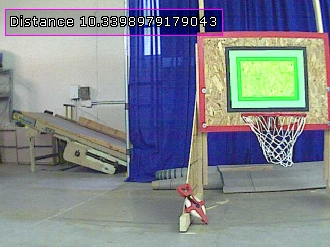This identifies the distance to the targets but not how to align towards the target. There are several ways to accomplish this depending on your robot configuration.

• Angular Measurement - The idea here is to calculate at what angle the robot or turret needs to turn in order to align to dead center of the target. This number can be calculated from the visual image and sent to a system that knows how to turn X number of degrees. This requirement of turning X degrees would need some form of sensor based feedback (like a Gyro or potentially Encoders) in order to know how far the system has currently turned before stopping. The issue with this system is that the robot may not be stationary or the system may not be very accurate.

The angle of rotation can be calculated by determining the number of pixels that equate to the width of the image and the field of view angular degrees. By knowing how many pixels off your target is from the center of the screen you can use the following formula to determine the relative amount of left or right rotation that would be needed to rotate the turret such that the target is dead center with respect to the camera.

```rotation_angle = (camera_field_of_view * (center_of_screen_x - center_of_target_x)) / image_width
```
So for example, the image below has an offset of 224 with a field of view of 47.5 degrees so the rotation would be
```rotation_angle = (47.5 * (224)) / 640
or
rotation_angle = 16.625 degrees to the left
```
• Distance Measurement - The idea here is to calculate the distance the robot would need to move to one side in order to align to the dead center of the target. This is possible with Mecanum drive wheels where the robot can crab to the right of left without needing to pivot. This calculation would instead require a distance left or right needed to move in order to best align the robot.
• Iterative Feedback - The final idea is more of an approach than a specific calculation. We can use the visual feedback to constantly tell us how to change our current state in order to achieve a better position. This method requires that the camera sensor be quickly processed and feed new information to actuators that will then update the robot position which will then again be processed by the camera in near real time. This iterative approach does not require any precise calculations that may or may not change during the competition due to worn equipment or lower battery levels.

We then proceed by discussing how to create the visual iterative feedback loop in order to align a robot with a visual target. Note that while we will assume angular changes this method will work just as well for crab like movement too.

If we have a look back at our original images we can calculate the center of the visual target with respect to the center of the image. For now we will assume that the center of the image is where we would like the robot to be before we execute a shoot basketball command.The images have been annotated to show the center of the target to the center of the screen with the X difference between those two points being the "Offset" variable shown. We can see that one is negative and one is positive relative to the center of the image. We can use this sign of the offset between these two points to determine which way the robot should move.

The next question is then by how much should the actuator move? The answer will depend on your hardware. Lets suppose that we are working with a turret based robot that has the turret moving based on a motor. We know what direction to make the motor spin but we don't know how much. The answer to this situation is more empirical (i.e. just test it) than mathematical (at least it is easier empirically). We first take the offset from screen center and multiply that by some factor. This factor should at first be a small number that should keep the maximum possible value (i.e. the width of the screen) within the allowed values for your motor. For example, if you motor PWM values range from 0 to 255 with 128 being neutral a good factor to start with would be 0.15 which would keep the maximum possible value based on a 640x480 image size to

```((640/2)*0.15)+128 = 176
```
This is how a pixel position can be converted into a motor value. Thus based on the above images the following results would be calculated:
```((224)*0.15)+128 = 161
((-197)*0.15)+128 = 98
```
Note that the second result is below 128 which moves the motor in the other direction.

So if we use these values and feed them into the motor controller to move the robot or turret the robot will start moving. This also includes the camera ... which is still active. As soon as the camera starts moving the image is updated and the angle that the target is off-center also changes. This change then immediately issues another motor value which will update the motor value quite quickly. This visual feedback loop will continue to constantly try to get the robot or turret positioned such that the target is dead center.

The value we used may cause your robot to move very slowly. Try increasing the factor from 0.15 to 0.2 or 0.3 and see the results. As you speed up the movement you will notice that at a certain speed it will start to oscillate around the center of the target. This is caused by a too high factor making the motor move too fast to stop at the exact center of the image. When this overshoots, the camera will then direct it back the other way ... which again may overshoot. If you see this oscillation you will need to reduce your factor back down to a point where it moves quickly but does not oscillate.

The speed of the visual feedback is what makes this system resilient to the choice of a factor that converts from pixel distance to motor speed.You don't need to know the exact number because the system will self correct to some degree. This system also has the advantage that it can be performed while the robot is moving and will keep track of the target assuming your mechanics can keep up with the changing signals.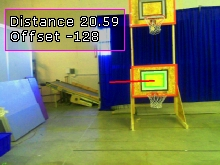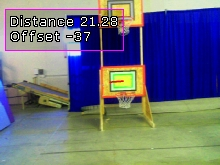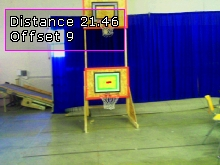Motor = 109 Motor = 122 Motor = 129 (overshot a little)

To try this out yourself:

3. Load and Run the angular measurementrobofile which shows angle of rotation to the target.
4. Load and Run the iterativerobofile which should produce the above results.